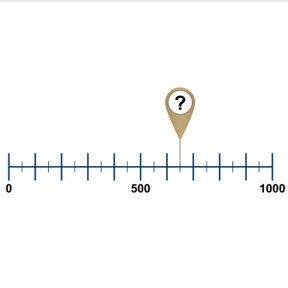Number line to 1,000 by 50s and 100s

# Number line to 1,000 by 50s and 100s

No account needed.8,000 schools use Gynzy92,000 teachers use Gynzy1,600,000 students use Gynzy

## General

Student learn to place 50s and 100s on the number line to 1,000. They learn that you can make jumps of 50 or 100.

2.NBT.A.2

## Relevance

Discuss with students that it is useful to be able to place a number on a number line, so you know where a number belongs. You can use this to determine if a number is bigger or smaller.

## Introduction

Write a number to 1,000 and show it to the class. Next the students are asked to write a number that jumps 100 forwards. Then skip count in 100s to 1,000. Write a new number, and have them write the next number that jumps 100 backwards. Next an empty number line is shown with a few arrows. The students must match the arrows to the given numbers. Before deciding what the numbers are, place the tens numbers on the number line.

## Development

Explain to the students that a long mark represents hundreds and a short mark represents fifties. Explain what hundreds and fifties are and explain that you count forwards from left to right. You can skip count in 50s or 100s. Practice this with the students and fill in the missing numbers. Tell students that they first should place hundreds on the number line, so you can see which number belongs where.

Check that students understand the number line to 1,000 in 50s and 100s by asking the following questions:
- How do you place a number on the number line?
- Where do you start counting?
- Which jumps can you make a on a number line?

## Guided practice

Students practice placing numbers on the number line. They first are given four answers to choose between, and then have to fill in the answer themselves.

## Closing

Discuss with students that it is important to know how to place numbers on the number line, because you then know where a number belongs. Repeat that the long mark represents hundreds and the short mark represents fifties. Play a game in which students point at a number on the number line and say the number. Are they saying the right number? Or are they trying to fool their classmates? HAve the class check what they say.

## Teaching tips

Students who have difficulty placing 50s and 100s on the number line to 1,000 can practice skip counting in 50s and 100s.

### The online teaching platform for interactive whiteboards and displays in schools

• Save time building lessons

• Manage the classroom more efficiently

• Increase student engagement